# xgboost中的数学原理

## CART回归树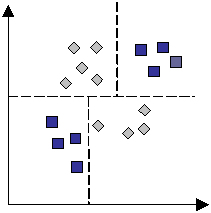CART决策树又分为回归树和分类树，CART回归树，它假设了决策树是一颗二叉树。它通过不断地将特征进行分裂(划分为左右两半)来构造一颗决策树。正是因为这一点，我们在使用boosting的时候，需要对离散变量进行one-hot编码处理。你可以想象一下，将一个离散的变量划分为两部分其实是完全没有意义的，因为离散的变量之间并不存在大小关系。

xiRm(yif(xi))2

R1(j,s)={x|x(j)s} and R2(j,s)={x|x(j)>s}

minj,s[minc1xiR1(j,s)(yic1)2+minc2xiR2(j,s)(yic2)2]

xgboost的建树过程其实是与CART回归树类似的，有了CART回归树的知识，我们理解xgboost的原理也可以轻松很多。接来下将会开始进入xgboost原理的主题。

## boosting介绍

boosting的核心思想就是我们希望训练出K颗树，将它们集成起来从而预测我们的Y。我们可以用以下公式表示：

y^=ϕ(xi)=k=1Kfk(xi)where F={f(x)=wq(x)}(q:RmT,wRT)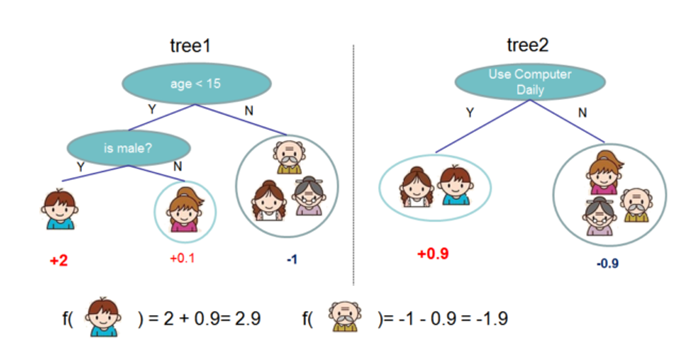## xgboost原理

L(ϕ)=il(yi^,y)+kΩ(fk)where Ω(f)=γT+12λ||w||2

L(t)=i=1nl(yi,yi^(t1)+ft(xi))+Ω(ft)

l=12(yf(x))2

gi=lf=f(xi)yihi=2lf2=1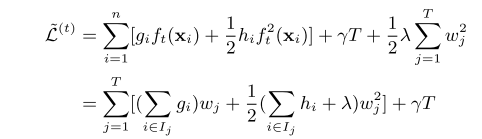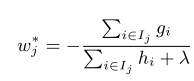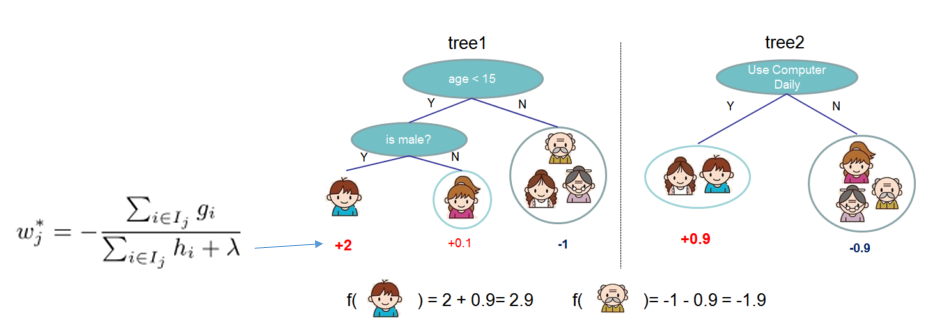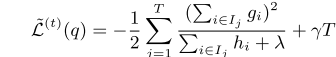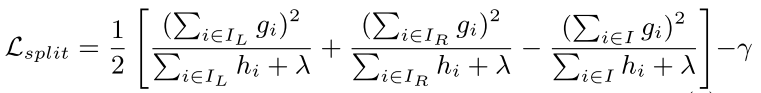## 建树时的终止条件

max_depth最大树深度：当树达到最大深度时则停止建立决策树。

min_child_weight:最小的样本权重和，样本权重和就是hi$\sum h_i$，当样本权重和小于设定阈值时则停止建树。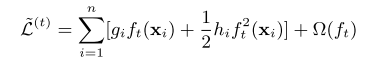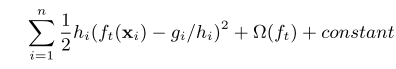## 样本权重

xgboost还支持设置样本权重，这个权重体现在梯度，和二阶梯度上，

grad_xgb[i] = grad[i] * case_weight[i]
hess_xgb[i] = hess[i] * case_weight[i]

# 参考文献

Chen, Tianqi, and Carlos Guestrin. “Xgboost: A scalable tree boosting system.” Proceedings of the 22Nd ACM SIGKDD International Conference on Knowledge Discovery and Data Mining. ACM, 2016.